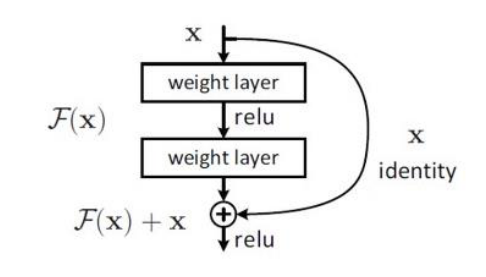# How to apply residual links to simple FFNN

Hi,
I want to apply the residual link used in ResNet to a simple model. Can I just add the input x and the output value passed through the linear layer in the element-wise direction as shown below?
Is there anything else I need to do more?

``````class neuralNet(torch.nn.Module):

def __init__(self, input_size, hidden_size, num_classes):
super(neuralNet, self).__init__()
self.linear1 = torch.nn.Linear(input_size, hidden_size)
self.relu1 = nn.ReLU()
self.linear2 = torch.nn.Linear(hidden_size, hidden_size)
self.relu2 = nn.ReLU()

self.linear3 = torch.nn.Linear(hidden_size, hidden_size)
self.relu3 = nn.ReLU()
self.linear4 = torch.nn.Linear(hidden_size, output_size)
self.relu4 = nn.ReLU()

def forward(self, x):
out = self.linear1(x)
out = self.relu1(out)
out = self.linear2(out)
out = out.add(x)            # H(x) = F(x) + x
out = self.relu2(out)

out = self.linear3(out)
out = self.relu3(out)
out = self.linear4(out)
out = out.add(x)            # H(x) = F(x) + x
out = self.relu4(out)

return out
``````Your approach should work and the `torchvision` implementation of `ResNet` uses a similar approach as seen here.

1 Like

Thank you always for good answers every time. It was very helpful.